Electron. J. Differential Equations, Vol. 2017 (2017), No. 292, pp. 1-11.

### Positive solutions for p-Laplacian equations of Kirchhoff type problem with a parameter Qi Zhang, Jianping Huang

Abstract:
In this article, we consider the existence and non-existence of positive solutions for the Kirchhoff type equation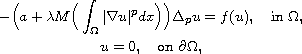where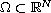is a bounded domain with a smooth boundary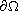, a is a positive constant,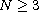,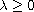,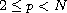, M and f are positive continuous functions. Under some weak assumptions on f, we show that the above problem has at least one positive solution whenis small and has no nonzero solution whenis large. Our argument is based on iterative technique and variational methods.

Submitted September 11, 2017. Published November 27, 2017.
Math Subject Classifications: 35B30, 35B09, 35J50, 35J62.
Key Words: Positive solution; p-Laplacian equation; iterative technique.

Show me the PDF file (256 KB), TEX file for this article.Qi Zhang School of Mathematics and Statistics Central South University Changsha, Hunan 410083, China email: zq8910@csu.edu.cn Jianping Huang School of Mathematics and Statistics Central South University Changsha, Hunan 410083, China email: huangjianping@csu.edu.cn